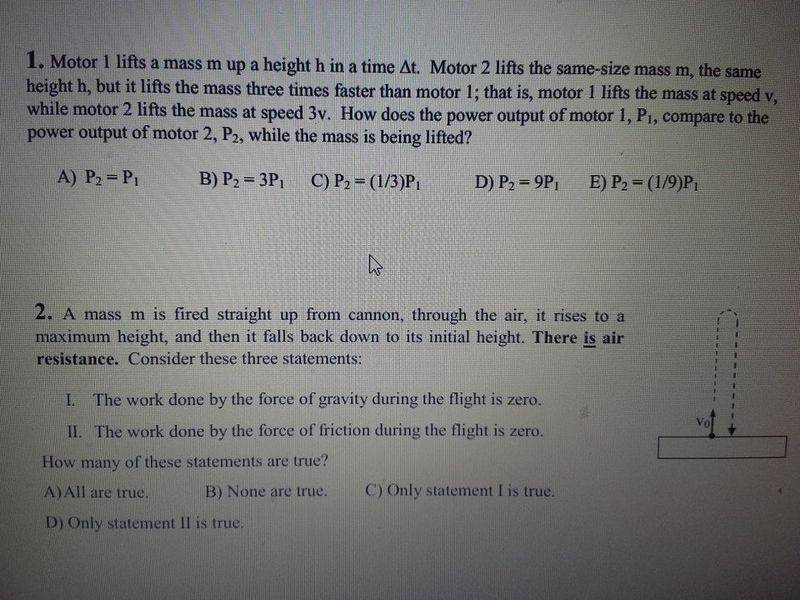Work, Gravity and air resistance question

Homework Statement

A mass m is fired straight up from a cannon, through the air, it rises to a maximum height, and then it falls back down to its initial height. There is air resistance. Consider these three statement.

I. The work done by the force of gravity during the flight is Zero.
II. The work done by the force of friction during the flight is zero.

How many of these statements are true?
A.) All are true./

B.) None are true.

C.) Only statement I is true

D) Only statement II is true.

Only statement II is true.Homework Equations

when a mass is brought to rest, the work done by the frictional force on it is equal to the kinetic energy of the object, KE=1/2 mv2. (friction) Newton’s universal law of gravitation:

F = Gm1m2/r2,
where F is the force due to gravity, between two masses (m1 and m2), which are a distance r apart; G is the gravitational constant.

The Attempt at a Solution

At first I thought statement C was true, but because When an object is falling freely, the force of gravity is doing work against the resistance from inertia and the air resistance or drag on the object. So I am thinking both statements are false because gravity is doing work against the resistance from inertia and the air resistance or drag on the object. The forces acting on the object are gravity and the opposite or resistive forces of inertia and air resistance.

Because the question is saying that both gravity and air friction arent doing any work when the object falls

i think none are true

and isnt air resistance kinetic energy? Thanks for your help! :)

Last edited:

Work done is always force into displacement. As the object comes back to it's initial position, there is 0 net work on the body. So, I guess there is no work done by either force on the body. I may be wrong though, I'm not sure.

Work done is always force into displacement. As the object comes back to it's initial position, there is 0 net work on the body. So, I guess there is no work done by either force on the body. I may be wrong though, I'm not sure.

Thanks for your input. I've came to the same conclusion to, but I'm confused about the "flight" part of the question.

The gravitational force first opposes the motion of the body(while the body is moving upwards) and then supports the motion while the body falls from the max height.So what will be the workdone by gravity in the whole trip?

Welcome to PF!

Last edited:
Let the maximum height be h

work done by gravity during upward flight = -mgh (-as force is opposite to displacement, cos180 = -1)
work done by gravity during downward flight = mgh

Net force = -mgh+mgh = 0

I don't know the exact expression for air resistance, but since it always acts against the direction of motion, it'll act downward during upward flight, and upward during the downward flight. My mind says it'll be zero.

yes it is correct for gravity.But air resistance opposes the motion of the mass irrespective of its direction.Hence it always does negative work which is not zero

So then the answer would be none are true since both gravity and air resistance are putting in work, right?

No see the post #4.Gravity does a negative work when the mass is going up and positive work when the mass is descending.So the net work will be zero.Are you getting it?

•1 person
So gravity itself cancels out, and that only leaves friction. So then I guess my answer would be C right?

right:)

•1 person
I really want to thank you for helping me. You guys are so awesome. My major is Computer Science but my minor is physics. I'm starting at the bottom, but again thank you all for tolerating me!! Michio kaku and Einstein came to me in a dream, and I hope the next post I make, will be the image of my BS degree!!! You guys are so awesome and thanks!!!!!

you are always welcome